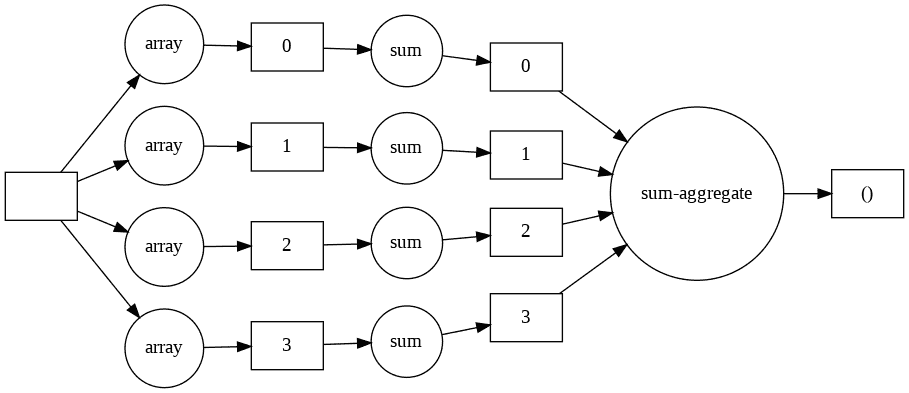# Beginner's Guide to Dask ArraysDevesh Vishwakarma Sept 26 2020 · 3 min read

### Introduction

When you begin your data science journey with python, you will quickly understand that Pandas and Numpy are the core libraries utilized for all sorts of data analysis and data manipulation. Any data analysis or data manipulation operation that you can think of is already implementable on pandas and numpy. The main reason that these libraries have been so popular is the fact that they combine high level usable and flexible API's with high-performance implementations and easy to use structures. Pandas and Numpy are incredible libraries, but they are not always computationally productive. Even these libraries have certain constraints in terms of scalability and speed when working on large datasets. As a solution to these issues, Dask comes in.

Dask is a flexible library for parallel computing in python. It is a python library that can deal with big datasets on a solitary CPU by utilizing multiple cores or on distributed machines. It works in good synergy with other python packages while giving them the versatility of parallel computing and scalability. The data structures available in dask includes:-

• Dask Arrays -  Dask arrays coordinate many Numpy arrays, arranged into chunks within a grid.
• Dask Dataframe - Dask DataFrame is a huge parallel DataFrame composed of many smaller Pandas DataFrames, split along the rows.
• Dask Bag - Dask Bag is a parallel list that can store any Python datatype with convenient functions that map over all of the elements.
• In this article, we'll have a close look at the working of dask array along with the numpy array for numeric processing.

Before exploring the dask array let's first look at how to set-up dask on your system. You can install dask with conda or with pip.

• Installation using conda
• ``conda install dask``
• Installation using pip
• ``python -m pip install "dask[complete]"``

Dask array provides a parallel, larger-than-memory, n-dimensional array using blocked algorithms. In simple words, its distributed numpy array.

• Parallel-It uses all cores for parallel computation
• Larger-than-memory-Lets you work on datasets that are larger than your available memory by breaking up your array into many small pieces, operating on those pieces in an order that minimizes the memory footprint of your computation, and effectively streaming data from disk.
• Blocked algorithms- Instead of operating on entire rows or columns of an array, blocked algorithms operate on submatrices or blocks i.e. Perform large computations by performing many smaller computations.
• ### Converting a Numpy array into Dask array

``import numpy as npimport dask.array as da``

We create a numpy array containing 10000 elements produced randomly.

``a=np.random.rand(10000)``

We can use the `from_array()` function of dask to convert a numpy array into a dask array. Additionally we have to define an argument` chunk `this is the number of elements in each piece.

``n_chunks=4a_dask=da.from_array(a,chunks=(len(a)/n_chunks))print(a_dask)``

Output

### Using Methods/Attributes with Numpy vs Dask

• using mean() in numpy
• ``#a=(10000,) size randomly assigned arrayprint('mean calculation done over numpy array:',a.mean())``

a.sum() computes the sum immediately.

• ``print(a_dask.mean())``

a_dask.mean() builds an expression of the computation don't do it yet

• ``print('mean calculation done over dask array:',a_dask.mean().compute())``

Dask array objects are lazily evaluated. Operations like .mean() build up a graph of blocked tasks to execute. We ask for the final result with a call to .compute(). This triggers the actual computation.

### Common Dask Array Methods/Attributes similar to Numpy

• Attributes: `shape`, `ndim` ,`nbytes`, `dtype`, `size`, etc.
• Aggregations: `max`, `min`, `mean`, `std`, `var`,` sum`, `prod`, etc.
• Array transformations: `reshape`, `repeat`,`stack`,`flatten`,`transpose`,`T`, etc.
• Mathematical operations: `round` , `real `, `imag` , `conj` , `dot` , etc.
• ### Working with chunks/parts in Numpy vs Dask

• Finding out the sum of all the elements of data by dividing it into small parts using numpy
• ``n_parts=4chunk_size=int(len(a)/n_parts)total=0for i in range(n_parts):  starting_index=int(i*chunk_size)  print('index of part '+str(i+1)+' start from '+str(starting_index))  a_parts=a[starting_index:starting_index+chunk_size]  total += a_parts.sum()print(total)``

We start by defining the number of parts to break down our array than we iterate over a for loop calculating the indices and slicing the array, adding them into a list, and summing up the elements partwise. Here each loop iteration is independent and can be executed in parallel.

Output-We get the end result as the sum of all the 10000 elements of array a.
• Using dask to find out the sum
• ``n_chunks=4a_dask=da.from_array(a,chunks=(len(a)/n_chunks))result=a_dask.sum()result.compute()``

We can see here we don't need to compute index and slice chunks explicitly. The dask array method does that for us. Calling just the `sum()` function builds an expression of the computation and the actual computation will be delayed until `compute()` is called.

• Visualizing the Task Graph using the `visualize` function.
• ``result.visualize(rankdir='LR')``The four rectangle in the middle represent our chunks of data as data flows from left to right, the sum method is invoked on each chunk.